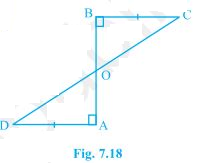# AD and BC are equal perpendiculars to a line segment AB (see Fig.) . Show that CD bisects AB.Solution

Given

AD and BC are equal perpendiculars to a line segment AB

To Prove

CD bisects AB

Proof

Two triangles ΔAOD and ΔBOC

∠ AOD = ∠ BOC {Vertically opposite angles}………………. (i)

∠ OAD = ∠ OBC {Given that they are perpendiculars}…. (ii)

AD = BC {As given}………………………………………………… (iii)

From above three equation both the triangle satisfies “AAS” congruency criterion

So, ΔAOD ≅ ΔBOC

AO and BO will be equal as they are corresponding parts of congruent triangles(CPCT).

So, AO = BO

Hence, CD bisects AB at O.